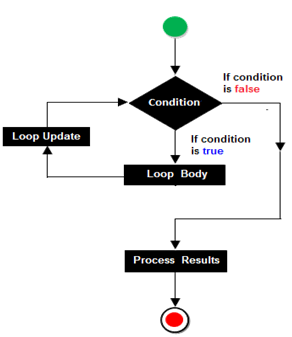#### While Loop

The while loop is entry controlled loop. The while loop keeps on executing the block of statement till the specified test condition evaluates to true. When the test condition becomes false, the program control is transferred out of the loop and passes to the statement after the body of the loop.

Syntax:`while(condition){	Statement 1;	Statement 2;	…………..	Statement n;}`

#### Example Programs

Program 

Write a program to input a number and print all its prime factors using prime factorization.

For Example,

INPUT: Enter an integer: 24

OUTPUT: Prime Factors using Prime Factorisation are:

2223

Program 

Write a program to input a number and check whether it is a Smith number or not. Smith number is such a number, the sum of whose digits equals the sum of the digits of its prime factors.

Smith number is a composite number in which the sum of its digits is equal to the sum of the digits of all its prime factors.

For Example 378 is a Smith Number as the sum of the digits of 378 are : 3+7+8 = 18. The prime factors of 378 are: 2, 3, 3, 3, 7 ( sum = 2+3+3+3+7 = 18).

Similarly 22 is a Smith Number as the sum of the digits are : 2+2=4. The prime factors of 22 are:

2 and 11 (Sum = 2+(1+1) = 4

Other Examples include 27, 58, 85, 94, 121, 166, 202, 265, etc.

Program 

Write a program to accept a number and check and display whether it is a spy number or not.

(A number is spy if the sum its digits equals the product of its digits.)

Example: consider the number 1124 , sum of the digits = 1 + 1 + 2 + 4 = 8

Product of the digits = 1 × 1 × 2 × 4 = 8

Program 

Write a Program in Java to input a number and check whether it is a Disarium Number or not.

Note: A number will be called DISARIUM if sum of its digits powered with their respective position is equal to the original number.

For example 135 is a DISARIUM (Workings 11+32+53 = 135, some other DISARIUM are 89, 175, 518 etc)

Sample:

Enter a Number : 135

135 is a Disarium Number.

Enter a Number : 219

219 is not a Disarium Number.

Enter a Number : 89

89 is a Disarium Number.

Program 

Write a Program in Java to input a number and check whether it is an Automorphic Number or not.

Note: An automorphic number is a number which is present in the last digit(s) of its square.

Example: 25 is an automorphic number as its square is 625 and 25 is present as the last digits

Sample:

Enter a Number : 25

25 is an Automorphic Number.

Enter a Number : 6

6 is an Automorphic Number.

Enter a Number : 9

9 is not an Automorphic Number.

Program 

Write a Program in Java to input a number and check whether it is a Fascinating Number or not.

Fascinating Numbers: Some numbers of 3 digits or more exhibit a very interesting property. The property is such that, when the number is multiplied by 2 and 3, and both these products are concatenated with the original number, all digits from 1 to 9 are present exactly once, regardless of the number of zeroes.

Let’s understand the concept of Fascinating Number through the following example:

Consider the number 192, 192 x 1 = 192 192 x 2 = 384 192 x 3 = 576

Concatenating the results : 192384576

It could be observed that ‘192384576’ consists of all digits from 1 to 9 exactly once. Hence, it could be concluded that 192 is a Fascinating Number.

Some examples of fascinating Numbers are : 192, 219, 273, 327, 1902, 1920, 2019 etc.

Sample Data:

Enter a number : 273

273 is a Fascinating Number.

Enter a number : 853

853 is not a Fascinating Number.

Enter a number : 95

Number should be of atleast 3 digits.

Program 

Write a Program in Java to input a number and check whether it is an Evil Number or not.

Evil Number: An Evil number is a positive whole number which has even number of 1’s in its binary equivalent.

Example: Binary equivalent of 9 is 1001, which contains even number of 1’s. A few evil numbers are 3, 5, 6, 9….

Design a program to accept a positive whole number and find the binary equivalent of the number and count the number of 1’s in it and display whether it is a Evil number or not with an appropriate message. Output the result in format given below:

Example 1

INPUT : 15

BINARY EQUIVALENT : 1111

NO. OF 1’s : 4

OUTPUT : EVIL NUMBER

Example 2

INPUT : 26

BINARY EQUIVALENT : 11010

NO. OF 1’s : 3

OUTPUT : NOT AN EVIL NUMBER

Program 

Write a java program to input any number and count the number of digits in number.

Program 

Write a java program to accept an integer number and print the frequency of each digit present in the number .

Sample:

Input : 3255435

Output:

Digit Frequency

2 1

3 2

4 1

5 3

Program 

Write a java program to accept an integer number to find out and print the difference between greatest and smallest digits presents in the number .

Program 

Write a Java Program which accept a binary number and print is equivalent Decimal Number.

`import java.util.*;class Binary2Decimal{   public static void main(String arr[])   {       Scanner sc=new Scanner(System.in);       System.out.println("Enter any Binary Number :");       int num=sc.nextInt();       int dec=0,c=0,r;       while(num!=0)       {           r=num%10;           dec=dec+r*(int)Math.pow(2,c);           num=num/10;           c=c+1;        }       System.out.println("The Decimal Equivalent ="+dec);   }}`